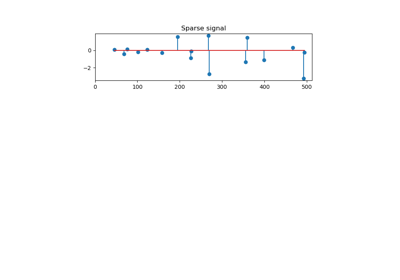# 3.2.4.1.8. sklearn.linear_model.OrthogonalMatchingPursuitCV¶

class sklearn.linear_model.OrthogonalMatchingPursuitCV(copy=True, fit_intercept=True, normalize=True, max_iter=None, cv='warn', n_jobs=None, verbose=False)[source]

Cross-validated Orthogonal Matching Pursuit model (OMP).

See glossary entry for cross-validation estimator.

Read more in the User Guide.

Parameters: copy : bool, optional Whether the design matrix X must be copied by the algorithm. A false value is only helpful if X is already Fortran-ordered, otherwise a copy is made anyway. fit_intercept : boolean, optional whether to calculate the intercept for this model. If set to false, no intercept will be used in calculations (e.g. data is expected to be already centered). normalize : boolean, optional, default True This parameter is ignored when fit_intercept is set to False. If True, the regressors X will be normalized before regression by subtracting the mean and dividing by the l2-norm. If you wish to standardize, please use sklearn.preprocessing.StandardScaler before calling fit on an estimator with normalize=False. max_iter : integer, optional Maximum numbers of iterations to perform, therefore maximum features to include. 10% of n_features but at least 5 if available. cv : int, cross-validation generator or an iterable, optional Determines the cross-validation splitting strategy. Possible inputs for cv are: None, to use the default 3-fold cross-validation, integer, to specify the number of folds. CV splitter, An iterable yielding (train, test) splits as arrays of indices. For integer/None inputs, KFold is used. Refer User Guide for the various cross-validation strategies that can be used here. Changed in version 0.20: cv default value if None will change from 3-fold to 5-fold in v0.22. n_jobs : int or None, optional (default=None) Number of CPUs to use during the cross validation. None means 1 unless in a joblib.parallel_backend context. -1 means using all processors. See Glossary for more details. verbose : boolean or integer, optional Sets the verbosity amount intercept_ : float or array, shape (n_targets,) Independent term in decision function. coef_ : array, shape (n_features,) or (n_targets, n_features) Parameter vector (w in the problem formulation). n_nonzero_coefs_ : int Estimated number of non-zero coefficients giving the best mean squared error over the cross-validation folds. n_iter_ : int or array-like Number of active features across every target for the model refit with the best hyperparameters got by cross-validating across all folds.

Examples

>>> from sklearn.linear_model import OrthogonalMatchingPursuitCV
>>> from sklearn.datasets import make_regression
>>> X, y = make_regression(n_features=100, n_informative=10,
...                        noise=4, random_state=0)
>>> reg = OrthogonalMatchingPursuitCV(cv=5).fit(X, y)
>>> reg.score(X, y)
0.9991...
>>> reg.n_nonzero_coefs_
10
>>> reg.predict(X[:1,])
array([-78.3854...])


Methods

 fit(X, y) Fit the model using X, y as training data. get_params([deep]) Get parameters for this estimator. predict(X) Predict using the linear model score(X, y[, sample_weight]) Returns the coefficient of determination R^2 of the prediction. set_params(**params) Set the parameters of this estimator.
__init__(copy=True, fit_intercept=True, normalize=True, max_iter=None, cv='warn', n_jobs=None, verbose=False)[source]
fit(X, y)[source]

Fit the model using X, y as training data.

Parameters: X : array-like, shape [n_samples, n_features] Training data. y : array-like, shape [n_samples] Target values. Will be cast to X’s dtype if necessary self : object returns an instance of self.
get_params(deep=True)[source]

Get parameters for this estimator.

Parameters: deep : boolean, optional If True, will return the parameters for this estimator and contained subobjects that are estimators. params : mapping of string to any Parameter names mapped to their values.
predict(X)[source]

Predict using the linear model

Parameters: X : array_like or sparse matrix, shape (n_samples, n_features) Samples. C : array, shape (n_samples,) Returns predicted values.
score(X, y, sample_weight=None)[source]

Returns the coefficient of determination R^2 of the prediction.

The coefficient R^2 is defined as (1 - u/v), where u is the residual sum of squares ((y_true - y_pred) ** 2).sum() and v is the total sum of squares ((y_true - y_true.mean()) ** 2).sum(). The best possible score is 1.0 and it can be negative (because the model can be arbitrarily worse). A constant model that always predicts the expected value of y, disregarding the input features, would get a R^2 score of 0.0.

Parameters: X : array-like, shape = (n_samples, n_features) Test samples. For some estimators this may be a precomputed kernel matrix instead, shape = (n_samples, n_samples_fitted], where n_samples_fitted is the number of samples used in the fitting for the estimator. y : array-like, shape = (n_samples) or (n_samples, n_outputs) True values for X. sample_weight : array-like, shape = [n_samples], optional Sample weights. score : float R^2 of self.predict(X) wrt. y.
set_params(**params)[source]

Set the parameters of this estimator.

The method works on simple estimators as well as on nested objects (such as pipelines). The latter have parameters of the form <component>__<parameter> so that it’s possible to update each component of a nested object.

Returns: self

## 3.2.4.1.8.1. Examples using sklearn.linear_model.OrthogonalMatchingPursuitCV¶Orthogonal Matching Pursuit